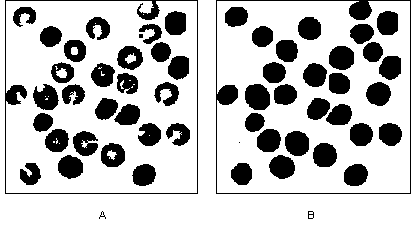NI Vision 2015 Concepts Help

Edition Date: June 2015

Part Number: 372916T-01

»View Product InfoDownload Help (Windows Only)

The advanced morphology operations are built upon the primary morphological operators and work on particles as opposed to pixels. Each of the operations have been developed to perform specific operations on the particles in a binary image.

## When to Use

Use the advanced morphological operations to fill holes in particles, remove particles that touch the border of the image, remove unwanted small and large particles, separate touching particles, find the convex hull of particles, and more.

You can use these transformations to prepare particles for quantitative analysis, observe the geometry of regions, extract the simplest forms for modeling and identification purposes, and so forth.

## Concepts

The advanced morphology functions are conditional combinations of fundamental transformations, such as binary erosion and dilation. The functions apply to binary images in which a threshold of 1 has been applied to particles and where the background is equal to 0.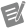Note  In this section of the manual, the term pixel denotes a pixel equal to 1, and the term particle denotes a group of pixels equal to 1.

### Border Function

The border function removes particles that touch the border of the image. These particles may have been truncated during the digitization of the image, and their elimination them helps to avoid erroneous particle measurements and statistics.

### Hole Filling Function

The hole filling function fills the holes within particles.

### Labeling Function

The labeling function assigns a different gray-level value to each particle. The image produced is not a binary image, but a labeled image using a number of gray-level values equal to the number of particles in the image plus the gray level 0 used in the background area.

The labeling function identifies particles using either connectivity-4 or connectivity-8 criteria. For more information on connectivity, refer to the connectivity section.

### Lowpass and Highpass Filters

The lowpass filter removes small particles according to their widths as specified by a parameter called filter size. For a given filter size N, the lowpass filter eliminates particles whose widths are less than or equal to (N – 1) pixels. These particles disappear after (N – 1) / 2 erosions.

The highpass filter removes large particles according to their widths as specified by a parameter called filter size. For a given filter size N, the highpass filter eliminates particles with widths greater than or equal to N pixels. These particles do not disappear after (N / 2 + 1) erosions.

Both the highpass and lowpass morphological filters use erosions to select particles for removal. Since erosions or filters cannot discriminate particles with widths of 2k pixels from particles with widths of 2k – 1 pixels, a single erosion eliminates both particles that are 2 pixels wide and 1 pixel wide.

The following table shows the effect of lowpass and highpass filtering.

Filter Size (N) Highpass Filter Lowpass Filter
N is an even number (N = 2k)
• Removes particles with a width greater than or equal to 2k
• Uses k – 1 erosions
• Removes particles with a width less than or equal to 2k – 1
• Uses k – 1 erosions
N is an odd number (N = 2k + 1)
• Removes particles with a width greater than or equal to 2k + 1
• Uses k erosions
• Removes particles with a width less than or equal to 2k
• Uses k erosions

Figure A represents the binary source image used in this example. Figure B shows how, for a given filter size, a highpass filter produces the following image. Gray particles and white particles are filtered out by a lowpass and highpass filter, respectively.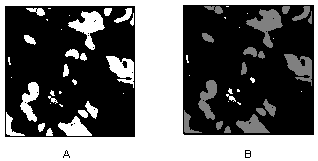### Separation Function

The separation function breaks narrow isthmuses and separates touching particles with respect to a user-specified filter size. This operation uses erosions, labeling, and conditional dilations.

For example, after thresholding an image, two gray-level particles overlapping one another might appear as a single binary particle. You can observe narrowing where the original particles have intersected. If the narrowing has a width of M pixels, a separation using a filter size of (M + 1) breaks it and restores the two original particles. This applies to all particles that contain a narrowing shorter than N pixels.

For a given filter size N, the separation function segments particles with a narrowing shorter than or equal to (N – 1) pixels. These particles are divided into two parts after (N – 1) / 2 erosions.

The above definition is true when N is an odd number, but should be modified slightly when N is an even number, due to the use of erosions in determining whether a narrowing should be broken or kept. The function cannot discriminate a narrowing with a width of 2k pixels from a narrowing with a width of (2k – 1) pixels, therefore, one erosion breaks both a narrowing that is two pixels wide as well as a narrowing that is one pixel wide.

The precision of the separation is limited to the elimination of constrictions that have a width smaller than an even number of pixels:

• If N is an even number (2k), the separation breaks a narrowing with a width smaller than or equal to (2k – 2) pixels. It uses (k – 1) erosions.
• If N is an odd number (2k + 1), the separation breaks a narrowing with a width smaller than or equal to 2k. It uses k erosions.

### Skeleton Functions

A skeleton function applies a succession of thinnings until the width of each particle becomes equal to one pixel. The skeleton functions are both time- and memory-consuming. They are based on conditional applications of thinnings and openings that use various configurations of structuring elements.

L-Skeleton uses the following type of structuring element:

 0 ? 1 0 1 1 0 ? 1

M-Skeleton uses the following type of structuring element:

 ? ? 1 0 1 1 ? ? 17

Skiz is an L-Skeleton performed on an inverse of the image.

#### L-Skeleton Function

The L-skeleton function indicates the L-shaped structuring element skeleton function. Using the source image in figure A, the L-skeleton function produces the image in figure B.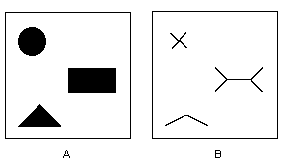#### M-Skeleton Function

The M-skeleton function extracts a skeleton with more dendrites or branches. Using the source image from figure A, the M-skeleton function produces the image shown in following figure.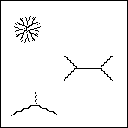#### Skiz Function

The skiz (skeleton of influence zones) function behaves like an L-skeleton function applied to the background regions instead of the particle regions. It produces median lines that are at an equal distance from the particles.

Using the source image from figure A, the skiz function produces the image in the following figure, which is shown superimposed on the source image.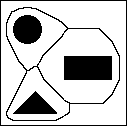### Segmentation Function

The segmentation function is applied only to labeled images. It partitions an image into segments that are centered around a particle such that they do not overlap or leave empty zones. Empty zones are caused by dilating particles until they touch one another.Note  The segmentation function is time-consuming. Reduce the image to its minimum significant size before selecting this function.

In the following figure, binary particles, which are shown in black, are superimposed on top of the segments, which are shown in gray shades.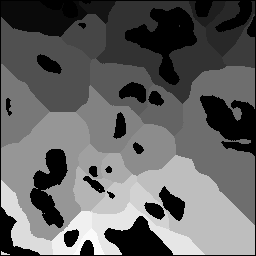When applied to an image with binary particles, the transformed image turns red because it is entirely composed of pixels set to 1.

#### Comparisons Between Segmentation and Skiz Functions

The segmentation function extracts segments that contain only one particle. A segment represents the area in which this particle can be moved without intercepting another particle, assuming that all particles move at the same speed.

The edges of these segments give a representation of the external skeletons of the particles. Unlike the skiz function, segmentation does not involve median distances.

You can obtain segments using successive dilations of particles until they touch each other and cover the entire image. The final image contains as many segments as there were particles in the original image. However, if you consider the inside of closed skiz lines as segments, you may produce more segments than particles originally present in the image. Consider the upper-right region in the following figure. This image shows the following features:

• Original particles in black
• Segments in shades of gray
• Skiz lines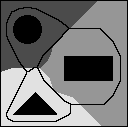### Distance Function

The distance function assigns a gray-level value to each pixel equal to the shortest distance to the particle border. This distance may be equal to the distance to the outer particle border or to a hole within the particle.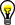Tip  Use the Danielsson function instead of the distance function when possible.

### Danielsson Function

The Danielsson function also creates a distance map but is a more accurate algorithm than the classical distance function. Because the destination image is 8-bit, its pixels cannot have a value greater than 255. Any pixels with a distance to the edge greater than 255 are set to 255.

For example, a circle with a radius of 300 yields 255 concentric rings whose pixel values range from 1 to 255 from the perimeter of the circle inward. The rest of the circle is filled with a solid circle whose pixel value is 255 and radius is 45.

Figure A shows the source threshold image used in the following example. The image is sequentially processed with a lowpass filter, hole filling, and the Danielsson function. The Danielsson function produces the distance map image shown in figure B.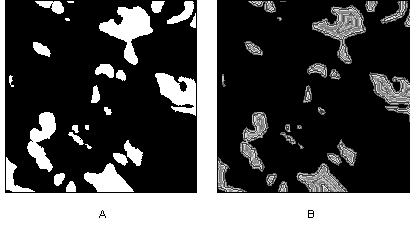View the resulting image with a binary palette. In this palette, each level corresponds to a different color. Thus, you easily can determine the relation of a set of pixels to the border of a particle. The first layer, which forms the border, is red. The second layer, closest to the border, is green, the third layer is blue, and so forth.

### Circle Function

The circle function separates overlapping circular particles using the Danielsson coefficient to reconstitute the form of an particle, provided that the particles are essentially circular. The particles are treated as a set of overlapping discs that are then separated into separate discs. This allows you to trace circles corresponding to each particle.

Figure A shows the source image for the following example. Figure B shows the image after the circle function is applied to the image.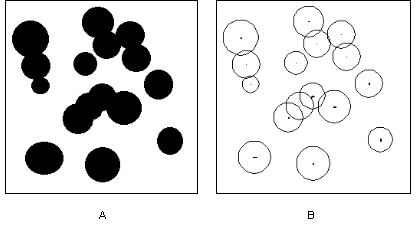### Convex Hull Function

The convex hull function is useful for closing particles so that measurements can be made on the particle, even when the particle contour is discontinuous.

The convex hull function calculates a convex envelope around each particle, effectively closing the particle. The image to which you apply a convex hull function must be binary.

Figure A shows the original labeled image used in this example. Figure B shows the results after the convex hull function is applied to the image.